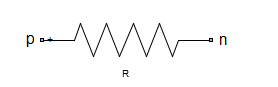# resistor

Resistor object

## Description

Use the `resistor` class to create a resistor object that you can add to an existing circuit.## Creation

### Syntax

``robj = resistor(rvalue)``
``robj = resistor(rvalue,rname)``

### Description

example

````robj = resistor(rvalue)` with a resistance of `rvalue` and default name, `R`. `rvalue` must be a numeric non-negative scalar.```

example

````robj = resistor(rvalue,rname)` creates a resistor object, `robj`, with a resistance of `rvalue` and name `rname`. `rname` must be a character vector.```

## Properties

expand all

Resistance value specified as a scalar in ohms.

Example: `50`

Example: `robj.Resistance = 50`

Name of resistor object, specified as a character vector. Two elements in the same circuit cannot have the same name.

Example: `'resis'`

Example: `robj.Name = 'resis'`

Names of the terminals of resistor object, specified as a cell vector. This property is read-only. The names `p` and `n` stand for positive and negative terminals, respectively.

Full path of the circuit to which the resistor object belongs, specified as character vector. This path appears only after the resistor is added to the circuit.

Note

"ParentPath" is only displayed after the resistor has been added into a circuit.

Circuit nodes in the parent nodes connect to resistor terminals, specified as a vector of integers. This property is read-only and appears only after the resistor is added to a circuit.

Note

"ParentNodes" are only displayed after the resistor has been added into a circuit.

## Object Functions

 `clone` Create copy of existing circuit element or circuit object

## Examples

collapse all

Create a resistor of resistance 100 ohms and display its properties.

```hR1 = resistor(100); disp(hR1)```
``` resistor: Resistor element Resistance: 100 Name: 'R' Terminals: {'p' 'n'} ```

Create an resistor object and extract the s-parameters of this resistor.

```hR = resistor(50,'R50'); hckt = circuit('example2'); add(hckt,[1 2],hR) setports (hckt, [1 0],[2 0]) freq = linspace (1e3,2e3,100); S = sparameters(hckt,freq); disp(S)```
``` sparameters: S-parameters object NumPorts: 2 Frequencies: [100x1 double] Parameters: [2x2x100 double] Impedance: 50 rfparam(obj,i,j) returns S-parameter Sij ```

Add a resistor to a circuit, display the parent path and parent nodes.

```hR = resistor(150,'R150'); hckt = circuit('resistorcircuit'); add(hckt,[1 2],hR) setports(hckt, [1 0],[2 0]) disp(hR)```
``` resistor: Resistor element Resistance: 150 Name: 'R150' Terminals: {'p' 'n'} ParentNodes: [1 2] ParentPath: 'resistorcircuit' ```

## Version History

Introduced in R2013b